# «GeOrigaMetry» An Approach to the Accessibility of Geometry for Blind People

«GeOrigaMetry» An Approach to the Accessibility of Geometry for Blind People
THIM-CHArt (UR-4004), University Paris 8, France

Page:
67-71
|
DOI:
https://doi.org/10.18280/mmc_c.811-412
27 June 2020
|
Accepted:
30 October 2020
|
Published:
31 December 2020
| Citation

OPEN ACCESS

Abstract:

Making geometry accessible for blind people, apart from the formal aspects, can pose some difficulties, especially in terms of accessibility to figures. To deal with this problem this article focuses on paper folding where both Euclidean and origami axiomatic systems are used simultaneously. In the first case, with a ruler and compass, we can solve quadratic problems in a plane. In addition, the axioms of origami allow us to address unanswered questions with classical geometry methods, which involve cubic equations, such as the trisection of an angle. An experiment with INJA (National Institute for Blind Youth, Paris) students and other blind people will take place so that we can see the possibilities offered by this method, which brings a ludic, but rigorous approach to these complex and frequently off-putting issues. We believe that this dynamic pedagogical approach can increase interest and motivation, encourage tactile stimulation and facilitate the development of specific structures of brain plasticity.

The article is written in a linear way, accessible to blind people; figures are provided to facilitate understanding for "visually impaired" people, who are not used to following a geometric concept without pictures. Finally, it should be noted that the method is particularly suitable in an inclusive education context.

Keywords:

accessibility of geometry, blind-people education, axiomatic systems and folding paper, tactile creativity, origami

1. Introduction

The creation of figures by folding paper (objects, animals, plants...) is a fairly widespread art with a very ancient origin, probably since the origin of paper in China in the second century [1, 2]. Two ancient traditions seem to be well established, with fairly specific bases, the Japanese «Origami» and the Spanish Papiroflexia , but it has spread widely and has become quite universal. In France it is widely represented by the MFPP (Mouvement Français des Plieurs de Papier) .

Pedagogical experiments using folding techniques have been offered for some time, in teaching environments for blind students as a tool for intellectual and playful development , taken up in France by M. Lucas in 2005 as part of the Blindmi project .

More recently, R.J. Lang’s and T. Gotani’s mathematical and more rigorous approaches have brought a formalism so that the development of a “geometry” of folding has emerged [7, 8], hence the title of “GeOrigaMetry”; while an interesting pedagogical focus [9, 10] has also emerged. These developments support the proposal presented in this article.

2. A Reminder of the Axiomatic System

Classical geometry is based on a well-known axiomatic system that allows a coherent development of science. By accepting and relying on these assumptions, it is possible to demonstrate theorems and build an exact science. We are used to accessing it from figures that facilitate the understanding of the corresponding elements, but graphic support is not strictly necessary.

In classical geometry these are the principles of the Euclidean axiomatic system . The concepts of dot, line, plane, angle, polygon, congruence, etc. are considered as known. The five basic assumptions are recalled below:

Axiom 1 (AE1): A straight line can be drawn from a point to any other point.

Axiom 2 (AE2): A finite straight line can be extended indefinitely.

Axiom 3 (AE3): A circle can be drawn, given a center and a radius.

Axiom 4 (AE4): All right angles are congruent. (All right angles are ninety degrees.)

Axiom 5 (AE5): If a straight line intersects two other straight lines such that the sum of the interior angles on one side of the intersecting line is less than the sum of two right angles, then the lines meet on that side and not on the other side (or postulate of parallels, in the version of Proclus: "If a straight line intersects one of two parallel lines, it will intersect the other also.").

In particular, the questioning of the latter postulate gave rise to non-Euclidean geometries, from the 19th century onwards.

Since the end of the last century we have been witnessing a theoretical development of folding techniques with a mathematical approach. This work is quite diverse: geometric , algebraic , topological, space geometry [14, 15], etc. Here we will address the areas closest to geometry that we believe are the most innovative for the accessibility of blind people.

Axioms of the origami of Huzita-Justin or Huzita-Hatori [7, 16, 17] are recalled below:

Axiom 1 (AO1): Given two distinct points p1 and p2, there is a unique fold that passes through both of them.

Axiom 2 (AO2): Given two distinct points p1and p2, there is a unique fold that places p1 onto p2.

Axiom 3 (AO3): Given two lines l1 and l2, there is a fold that places l1 onto l2. (This fold is not necessarily unique, especially if the intersection of the two lines is on the paper. Indeed, two segments of the same line can define two different folds (two bisectors).)

Axiom 4 (AO4): Given a point p1 and a line l1, there is a unique fold perpendicular to l1 that passes through point p1.

Axiom 5 (AO5): Given two points p1and p2 and a line l1, there is a fold that places p1 onto l1 and passes through p2.

Axiom 6 (AO6): Given two points p1 and p2 and two lines l1 and l2, there is a fold that places p1 onto l1 and p2 onto l2.

There is a seventh axiom (AO7), stated by Jacques Justin in 1989: “Given one-point p and two lines l1 and l2, there is a fold that places p onto l1 and is perpendicular to l2” .

It is possible to find a parallel between the first five axioms and the Euclidean axioms, or even construction facilities, for example (AO3) allows us to construct a bisector without having to access the top of an angle. But constructions derived from the axiom 6 (AO6) are not possible with a ruler and compass (except in particular cases). This assumption will allow us to solve third-degree equations, demonstrated by Justin  or solve problems like the sorting of an angle, as we will see later.

3. Some Practical Approaches for Pedagogical Experimentation

We discuss a few educational examples that will be put into practice during our experimentation.

The text will be used to make the folding process accessible to blind people (images are presented to facilitate access to people who can see).

We will always start from a square sheet on the unit side. We have four points; they are the four corners.

They are called A, B, C, D, clockwise:

Let's apply the first axiom (AO1): “Given two distinct points p1 and p2, there is a unique fold that passes through both of them”.

Consider two opposite corners AC (or BD). The folds that pass through AC or BD respectively, correspond to the diagonals of the square.

## 1.png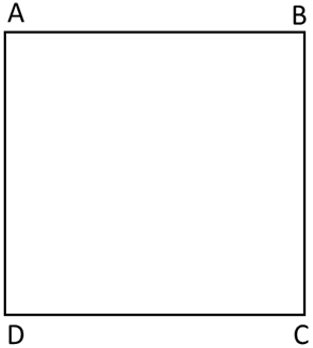We can also apply (AO2): “Given two distinct points p1and p2, there is a unique fold that places p1 onto p2”, bringing point B onto D (or A onto C respectively) and get the same result.

It is also possible to apply (AO3): “Given two straight lines l1 and l2, there is a fold that places l1 onto l2”. By using two adjacent sides we can achieve the same result.

## 2.png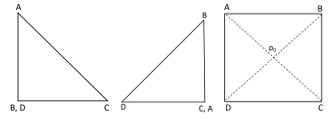With the intersection of these two diagonal folds, we get a new point p0 that corresponds to the center of the square. The size of the diagonals will be equal to square root of two (21/2).

3.1 Demonstration of the sum of the angles of a triangle

We now start from a triangular sheet whose peaks are ABC and the angles α, β and γ respectively.

1. With axiom 4 (AO4): “Given a point p1 and a straight line l1, there is a unique fold perpendicular to l1 that passes through point p1”. We will make a fold corresponding to the height of the triangle that runs from the vertex A to the opposite side BC. For this, we make a fold that goes through A and makes the side BC coincide with itself. We get A' in the side BC. The triangles AA'C and AA'B are right-angled.

## 3.png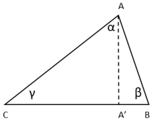2. With (AO2) we make the fold that brings A on A'. This fold defines M1, on the side AC, and M2on the side AB.

## 4.png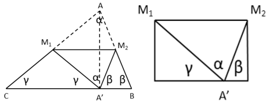3. The CA'M1and BA'M2triangles are isosceles. The angles α, β and γ coincide in point A'; the sum of them is 180 degrees.

Note that by folding the peaks of the two isosceles triangles, we obtain a rectangle whose area is the half of the original triangle; if we accept that the area of a rectangle is equal to the width multiplied by the length, then we can demonstrate that the area of a triangle (S) is half of the product of the base (B) by the corresponding height (H): 1/2S =1/2B x 1/2H; and S=1/2 B x H.

3.2 Construction of an equilateral triangle from a square (A, B, C, D):

1. To obtain the mediator of the square (AO2 or AO3), make a fold that joins AD and BC and defines p1 (middle of the side AB) and p2 (middle of the side CD).

2. Make a fold that passes through C and brings the corner B to the line p1, p2(AO5). We obtain the point E in the p1p2 segment. CE = BC

## 5.png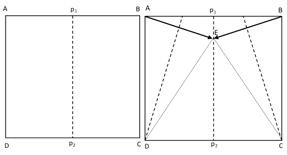3. Make the fold that goes through D and brings A to the linep1, p2, it coincides naturally with point E and DE = AD.

4. The triangle DCE is equilateral with both sides equal to the side of the square. The height of this triangle is (3/4)1/2 or 3 1/2/2, (square root of 3 divided by two).

Build the equilateral triangle inscribed in the square

In the earlier problem, the fold that brings B to the point E intersects with AB at p3.

The angle BC p3is 15 degrees [(90-60) /2].

## 6.pngLet us make the fold to obtain the other mediator of the square and trace the symmetrical fold such that C passes through and bring us D in this new mediator. This fold cuts AD in p4. The angle p4Cp3is 60 degrees; Cp4 = Cp3; and the triangle p4Cp3is equilateral, inscribed in the square. This triangle is maximum.

3.3 Haga's theorems

Haga's theorems (2008)  are interesting from an algebraic, geometric and pedagogical point of view.

1. Make a fold to obtain a mediator and the point M, at the center of the side AB (AO2 or AO3).

## 7.png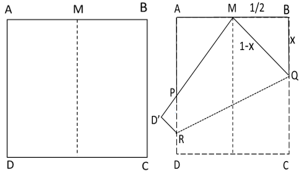2. Bring the corner C to Point M (AO2). With this fold, we get Q at the intersection with the side BC and R at the side AD. QC=QM. Moreover, the side DC side, after folding, crosses the AD side in point P. The corner D becomes D', after folding, and RD = RD’.

3. Theorem: The right-angled triangles MBQ, APM and D'PR are Pythagorean (side ratio: 3, 4, 5).

Demonstration: consider the side of the square. In the right-angled triangle MBQ, MB=1/2; BQ=x; QM=1-x, then x2=(1-x)2 - 1/4 and BQ=3/8; MB=4/8 and QM=5/8. In addition, the right-angled triangles MBQ, APM and D'PR are similar (equal angles by orthogonal sides) and therefore have the same ratios. Note that AP=2/3.

4. The perimeters (Per) of these three triangles have the following relationship:

Per(D'PR) +Per(BMQ)= Per(APM) (the sum of the perimeter of the two smaller triangles is equal to the perimeter of the larger one).

5. Demonstration: The anterior theorem allows us to calculate the sides of the three triangles by proportionality. We get the values AP=2/3, PD=1/3 and:

(3/24+4/24+5/24) + (3/8+4/8+5/8) = (3/6 +4/6+5/6)

Note that this last property is also valid if the M point is not in the center of the AB side, but the demonstration is more complex. Note that the sum of the perimeters of the three triangles will always be equal to the perimeter of the square (if the square side is equal to the unit, the sum of the perimeters of the three triangles will always be equal to 4) and that the three triangles are always similar. One can also refer to “Affaire de logique” nr.1138 (in French) .

3.4 Trisection from an acute angle 

This is one of the classic problems of ancient Greece (such as squaring the circle or duplicating the cube). After unnecessary attempts for more than two thousand years and millions of hours of struggle to find a solution to these problems, the theorem of Wantzel  demonstrated in 1837 that this was impossible (except in special cases) with a ruler and compass.

Axiom 6 (AO6):Given two points p1 and p2 and two straight lines l1 and l2, there is a fold that places p1 onto l1 and p2 onto l2” brings building elements that do not exist in Euclidean geometry and solves some problems whose non-feasibility criteria is demonstrated by Wanzel. Here we discuss the classic case of dividing an angle.

This construction for sharp angles is due to Abe . A folding solution for obtuse angles was also proposed by Jacques Justin in 1984 .

As in previous cases, we will begin from a square sheet (ABCD). The process is as follows:

1. Let's make any fold that goes through D and the straight line on the top of the square (AB). This fold, with the CD side, defines the angle α, that we want to divide into three equal parts. This fold cuts AB at the point E.

## 8.png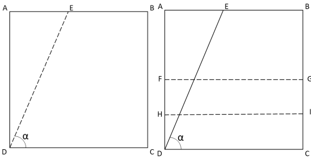2. Let us make a fold parallel to CD (preferably at or above the mediator to facilitate folding). We get the point F in the side AD and the point G in the side BC.

3. Let us bring the side CD to the line FG, (AO3), the fold thus obtained gives us H on AD and I on BC. By construction we have FH=HD=GI=IC.

4. By applying (AO6) we bring the vertex D on the HI line to get the D' and the F-point on the line DE to get the point F'. The line F'D cuts the side AD at point J. The fold thus obtained (JL) is a bisector of the angle DJD'. This fold cuts HI at K and DC at L. The point H becomes H' on the line JD.

## 9.png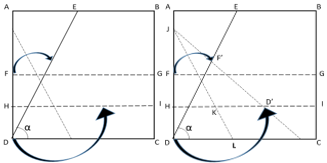5. We fold now by DK and DD' (AO1). We divide the angle α into three parts F'DH', H'DD' and D'DC. Now we have to demonstrate that these angles are equal.

## 10.png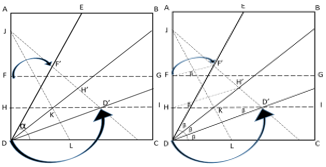6. Demonstration: The triangle DD'K is isosceles, by symmetry (DK = KD'). The angles KD'D and KDD' are equal (β). The angles KD'D and D'DC are equal (alternate-internal), so H'DD'=D'DC=(β). In addition, FH = HD = F'H' = H'D' and F'DD' is isosceles with height = DH', so F'DH'=H'DD' (β) Q.E.D. (It is also possible to use the parallelism of FF'HH' DD' and the isosceles triangle KHH').

4. Experimentation

4.1 Type of paper

A first experiment was carried out to find the most ergonomic type of paper for easy access to folding by blind people. We tested different grammages (60, 80, 100, 120, 160 g/m2) but also the type of paper: “sandwich” (two tissue papers glued to a metallic paper), elephant, verged, double texture, etc. It seems that the most suitable, at least initially (without taking into account a possible adaptation by learning), is between 120 and 160 g/m2, which allows a better access to the marking of the folds and their intersections, without limiting too much the ease of folding. However, this limits the number of folds and it is contradictory to the “theoretical” approach that considers a paper without thickness. The size of the paper is also a factor to be taken into account. In general, it will depend on the overlapping of the folds and the complexity of the folding to be developed. We used 9x9cm and 21x21cm squares. It is possible that, at least initially, in an educational context, pre-marking the paper facilitates folding, especially if the folds are complex such as the trisection of the angle.

4.2 Accessibility and acceptance by blind students

(The planned protocol was interrupted by the closure of the INJA as a result of containment measures due to the Covid-19 pandemic).

The experiment involves some folding sessions with students (from the third to the senior year) and blind people to study the accessibility to the origami axioms and to each of the geometrical developments that appear in this document. To date, the constructions described show the following results:

In general, the progress of the abovementioned constructions seems to be very well adapted.

AO1, AO2, AO3 axioms do not pose any accessibility problem if they are sides or points defined on sides by an intersection with a crease. Neither are AO4 and AO5 if the lines involved are expressed as folds.

The sum of the angles of a triangle; the construction of an equilateral triangle (in both versions) and Haga's theorem seem to be easily accessible.

In the trisection of an angle: practical problems have arisen for the tactile highlighting of the points F', D' and K in order to facilitate folding at these points. It is a problem of detecting projection points on a surface. One possibility is to formalize the line projections with folds and detect the intersection points of these folds; another simple possibility would be to work on a soft surface (cork, silicone) that can be used to nail pins, or mark points with embossing, to allow the detection of projection points. But practice should permit the student to advance fairly quickly.

5. Conclusion

Paper folding, as well as the recent axiomatic system associated with it, seem to be a good approach to geometry for blind people. Touch-based manipulation of the paper folding procedures seems to be compatible with the disability considered. We found a small initial difficulty when applying it to axioms that involve intersections (projections) of points with straight lines (inside the paper) or straight lines on unfolded straight lines (AO5, AO6). But we noticed rapid progress with practice.

Moreover, in a context of inclusive pedagogy, the method can be considered as a ludic incentive that stimulates communication between blind and non-blind students.

Acknowledgments

The author would like to thank Josephine Lahmani for her reading review and verification of the ratings (without access to the images!), Peter Wagstaff, Isis Truck, Susana Herrera and the STUDENTS of INJA, who were very motivated to participate in the experiments, as well as their mathematics teachers Samuel Huyet-Blau, Amina Abaoui and Christine Enault.

References

 Palacios, V. (2008). Historia de la Pajarita. Barcelona.

 Wang-Iverson, P., Lang, R.J., Mark, Y.I.M. (2011). Origami 5: Fifth International Meeting of Origami Science, Mathematics, and Education. CRC Press.

 de Unamuno, M. (1969). Apuntes para un tratado de cocotología. Almacenes Generales del Papel.

 French Movement of Paper Folds. Association 1901. 56 Coriolis Street, Paris 75012.

 Kase, S. (1991). My origami teaching method for the physically disabled (including the blind) and the teacher's attitude. Proceedings of COET91, Birmingham, pp. 242-247.

 Mr. Lucas. (2005). «Teaching Origami. The Blind project» The Valentin Hay, No. 79, pp. 38-39.

 Lang, R.J. (2011). Origami Design Secrets. Mathematical Method for an Ancient Art. CRC Press.

 Gotani, T. (2019). Origamix. Theory and Challenges. The Grésivaudan Workshop.

 Garrido, M.B. (2015). Orisangakus, Desafíos Matemáticos con Papiroflexia. Real Soc. Matemotica Espa'ola and SM. Madrid.

 Pope, S., Lam, T.K. (2011). Origami and learning mathematics. Origami, 5, 205-218.

 Euclid of Alexandria, «The Elements» Vol 1, Books I-IV, PUF, Paris 1990, p531.

 Geretschlger, R. (2008). Geometric Origami. Arbelos Publishing, Shipley, UK.

 Hull, T. (2013). Project Origami, Activities for Exploring Mathematics. CRC Press.

 Simon, L., Arnstein, B., Gurkewitz, R. (1999). Modular Origami Polyhedra. Revised and Enlarged Edition Paperback.

 Loper, B. (2016). Modular Origami: The Art of Polyhedral Paper Folding: Use Origami Math to fold Complex, Innovative Geometric Origami Models Paperback. Tuttle Publishing.

 Huzita, H. (1989). Axiomatic development of origami geometry. Proceedings of the First International Meeting of Origami Science and Technology, 143-158.

 de la Peña Hernandez, J. (2001). Matemáticas y papiroflexia. AEP, Madrid.

 Justin, J. (1989). Résolution par le pliage de l’équation du troisieme degré et applications géométriques. Proceedings of the First International Meeting of Origami Science and Technology, Ferrara, Italy, pp. 251-261.

 Haga, K. (2008). Origamics: Mathematical explorations through paper folding. World Scientific.

 « Le Monde » n°23392 du 25 mars 2020, p24. Solution dans le n°du 1er avril 2020, p 24.

 Abe, H. (1984). The Mathematics of Origami. British Origami.

 Wantzel, P.L. (1837). Recherches sur les moyens de reconnaître si un problème de géométrie peut se résoudre avec la règle et le compas, Journal de mathématiques pures et appliquées, 1(2): 366-372.

 Fusimi, K. (1980). Trisection of angle by Abe. Saiensu supplement, 8.

 J. Justin. (1984). «Triseccion Angulo Obtus» described in British Origami, no. 107, pp. 14-15.